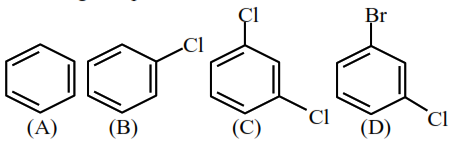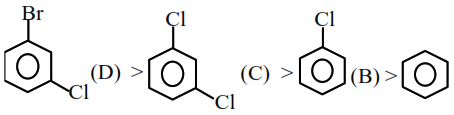# The correct decreasing`
Question:

The correct decreasing order of densities of the following compounds is :1. $(\mathrm{D})>(\mathrm{C})>(\mathrm{B})>(\mathrm{A})$

2. $(\mathrm{C})>(\mathrm{D})>(\mathrm{A})>(\mathrm{B})$

3. $(C)>(B)>(A)>(D)$

4. $(\mathrm{A})>(\mathrm{B})>(\mathrm{C})>(\mathrm{D})$

Correct Option: 1

Solution:

The density order